# Describing the translation and rotation of a square frame

• Igor Oliveira

## Homework Statement

Four equal discs of mass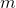ocuppy the vertices of a square frame made by four rigid bars of lengthand negligible mass. The frame is at rest on a horizontal table, and it can move with negligible friction. An instantaneous impulse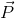is transmitted to one of the masses, in the direction of one of the squares diagonals. Describe completely the subsequent movement of the system.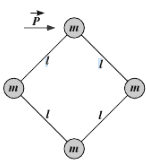Answer: The center of mass moves with constant velocity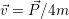and the frame spins with angular velocity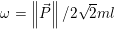## Homework Equations

Linear momentum: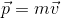Angular momentum: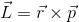Impulse-momentum theorem: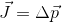## The Attempt at a Solution

I obtained the first result by assuming that the the linear momentum transmitted by the impulse will be divided into four chunks, which will be associated with each mass. Then, the center of mass will notably move with velocity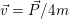. But why can I assume that? And shouldnt the velocity be less than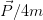, considering that part of the energy given to the frame will be associated with its rotation?

(This problem was found in a textbook written in portuguese, so the translation might not be the best. Please comment any doubt with the problem statement!)

#### Attachments

Ok, the total movement is the displacement of center or mass plus the rotation of mass around it.

How can you compute, the angular velocity ##\omega##?
Can you based in the geometry compute L? (p is totally transmited)
And the relation betwenn L, I and w?
Can you compute I for this system?

•Igor Oliveira
Thanks for the comment, Alejandro! I stopped thinking in terms of energy, and was able to solve this problem fairly easily.
(The terms in bold are vectors)
I) After the instantaneous impulse, there will be no net external force acting on the system. Thus, the linear momentum must be conserved. Besides that, we know that the center of mass (CM) moves as if all linear momentum was concentrated in it.

P = 4mvCM ⇔ vCM = P/4m.

II) On the other hand, after the instantaneous impulse, there will be no net external torque acting on the system. Therefore, the angular momentum must be conserved. Let ri be the position of mass i in relation to the CM, and vi its velocity. Then, we must have:

L = r1×(mv1) + r2×(mv2) + r3×(mv3) + r4×(mv4)
The direction of all vectors is the same. Then, we can work with the magnitudes of the vectors. Moreover, we know that the magnitude of ri is r, and the magnitude of vi is v.
L = rmv + rmv + rmv + rmv ⇔ L = 4mvr.
v = ωr ⇒ L = 4mwr2

But L = rP ⇒ rP = 4mwr2 ⇔ ω = P/4mr.
Also, r = 2⋅l/2. And finally: ω = P/22ml

Yes, I've made the problem, and only a half of A4 it's necessary for goal the solution